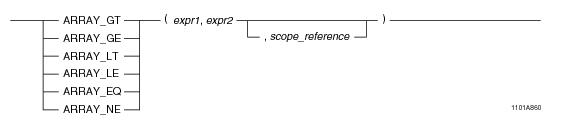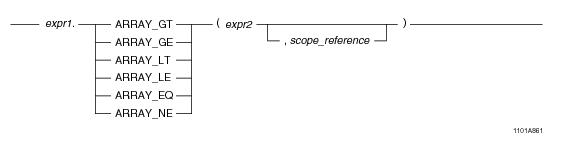# 15.00 - ARRAY Comparison Functions - Teradata Database

## Teradata Database SQL Functions, Operators, Expressions, and Predicates

Product
Release Number
15.00
Content Type
Programming Reference
Publication ID
B035-1145-015K
Language
English (United States)

## ARRAY Comparison Functions

### Purpose

Performs comparisons on the individual elements of an ARRAY value, whether referred to in its entirety or over a scope reference.

### Syntax

System function syntax:Method-style syntax:where:

 Syntax element… Specifies… expr1 an ARRAY expression or a scalar expression. An ARRAY expression is one of the following: A reference to an ARRAY column An ARRAY constructor expression. See “ARRAY Constructor Expression” on page 195. A UDF expression A UDM expression The element type of the array must be a numeric data type. expr2 an ARRAY expression or a scalar expression. An ARRAY expression is one of the following: A reference to an ARRAY column An ARRAY constructor expression. See “ARRAY Constructor Expression” on page 195. A UDF expression A UDM expression The element type of the array must be a numeric data type. scope_reference an optional ARRAY scope reference. See “ARRAY Scope Reference” on page 198.

### ANSI Compliance

This is a Teradata extension to the ANSI SQL:2011 standard.

### Argument Type

Either expr1 or expr2 or both arguments must be an ARRAY expression. For details, see “Usage Notes”.

### Supported ARRAY Comparison Functions

The following table provides a description of the supported ARRAY comparison functions.

 Relational Function Description ARRAY_GT Greater than function. Compares two expressions and determines whether expr1 is greater than expr2. If it is, the function returns a non-zero value in the corresponding result ARRAY element. If expr1 is less than or equal to expr2, the function returns zero in the corresponding result ARRAY element. ARRAY_GE Equal or greater than function. Compares two expressions and determines whether expr1 is greater than or equal to expr2. If it is, the function returns a non-zero value in the corresponding result ARRAY element. If expr1 is less than expr2, the function returns zero in the corresponding result ARRAY element. ARRAY_LT Less than function. Compares two expressions and determines whether expr1 is less than expr2. If it is, the function returns a non-zero value in the corresponding result ARRAY element. If expr1 is greater than or equal to expr2, the function returns zero in the corresponding result ARRAY element. ARRAY_LE Equal or less than function. Compares two expressions and determines whether expr1 is less than or equal to expr2. If it is, the function returns a non-zero value in the corresponding result ARRAY element. If expr1 is greater than expr2, the function returns zero in the corresponding result ARRAY element. ARRAY_EQ Equality function. Compares two expressions and determines whether expr1 is equal to expr2. If it is, the function returns a non-zero value in the corresponding result ARRAY element. If expr1 is not equal to expr2, the function returns zero in the corresponding result ARRAY element. ARRAY_NE Non-equality function. Compares two expressions and determines if expr1 is not equal to expr2. If the two expressions are not equal, the function returns a non-zero value in the corresponding result ARRAY element. If expr1 is equal to expr2, the function returns zero in the corresponding result ARRAY element.

### Usage Notes

For the relational functions ARRAY_GT, ARRAY_GE, ARRAY_LT, ARRAY_LE, ARRAY_EQ, and ARRAY_NE, two forms are supported for ARRAY types.

In the first form, both left-hand side (LHS) and right-hand side (RHS) operands are two instances of the same ARRAY type.

The relational operation is performed in a pairwise fashion, one by one for each matching pair of elements in the two arguments. If the optional argument scope_reference is defined, then the operation is performed only over the elements within the scope. All elements outside the boundaries of scope_reference are set to NULL.

The result is an ARRAY of the same type as the input array arguments, with three possible values: 1 (true), 0 (false), or NULL for all numeric types except DECIMAL(m,n) where m=n. For DECIMAL(m,n) where m=n, the function cannot store a value of 1 because no digits are permitted to the LHS of the decimal point. In this case, the function returns .9. Any higher level of precision above 1 is padded with zero to the right.

In the second form, one of either LHS or RHS is an ARRAY with a valid Teradata data type, and the other operand is a numeric value of the same data type as the one defined for the ARRAY in LHS.

The relational operation is performed by applying the numeric value argument to each element of the ARRAY argument. If the optional argument scope_reference is defined, then the operation is performed only over the elements within the scope. All elements outside the boundaries of scope_reference are set to NULL.

The result is an ARRAY of the same type as the input array argument, with three possible values: 1 (true), 0 (false), or NULL for all numeric types except DECIMAL(m,n) where m=n. For DECIMAL(m,n) where m=n, the function cannot store a value of 1 because no digits are permitted to the LHS of the decimal point. In this case, the function returns .9. Any higher level of precision above 1 is padded with zero to the right. If RHS evaluates to NULL, then NULL is returned.

For both forms of the relational comparison functions, if a NULL element is encountered in an ARRAY argument, the resulting ARRAY will contain a NULL element in that position. Comparison involving arrays are not affected by the optional DEFAULT NULL clause in the CREATE TYPE statement. However, the probability of getting an error is increased when comparing arrays that have been created without the DEFAULT NULL clause.

If an ARRAY argument contains any elements that are in an uninitialized state, an error is returned. Use a scope reference to avoid referencing a range of the ARRAY that contains uninitialized elements, or set any uninitialized elements to NULL. You can do this with the OEXTEND method. See “OEXTEND” on page 272.

### Restrictions

You cannot make relational comparisons of ARRAY values. You cannot use any of the relational comparison operators on ARRAY data. You can only make relational comparisons on individual elements of an ARRAY.

### Example

Consider the following 1-D ARRAY data type and table.

`CREATE TYPE item_price AS DECIMAL(7,2) ARRAY;`
` `
`CREATE TABLE inventory (itemkind INTEGER, `
`                        regular_price item_price,`
`                        sale_price item_price);`

Assume the following element values for the sale_price and regular_price arrays:

`sale_price[1:10] =  50, 100,  200,  90,  250, 550, 200, 200,  50,  75`
`regular_price[1:10] = 100, 300, 230, 110, 500, 550, 200, 400, 100, 150`

The following query returns a 1-D ARRAY with scope reference [1:10] of BYTEINT element type. During evaluation, each element within the specified scope, [5:10], in the regular_price array is compared with the corresponding element of the sale_price array using the relational function ARRAY_GT. The resulting values in the output array are 0 or a non-zero number for the elements within the scope reference, and NULL for all other elements of the array.

`SELECT ARRAY_GT(regular_price, sale_price, 5,10)`
`FROM inventory;`

The output from the query is a 1-D ARRAY with the following values:

`output_array[1:10] = [ NULL, NULL, NULL, NULL, 1, 0, 0, 1, 1, 1]`

The following query returns a 1-D ARRAY with scope reference [1:10] of type item_price. During evaluation, each element within the specified scope [5:10] in the regular_price array is compared with the corresponding element of the sale_price array using the relational function ARRAY_GT. The resulting ARRAY of element type BYTEINT value (0 or non-zero number) is multiplied by the corresponding element value of the regular_price array.

`SELECT ARRAY_MUL(regular_price, ARRAY_GT(sale_price, regular_price, 5, 10)) `
`FROM inventory;`

The output of the query is a 1-D ARRAY with the following values:

`output_array[1:10] = [ NULL, NULL, NULL, NULL, 500, 0, 0, 400, 100, 150]`

In the following query, the relational function ARRAY_LT compares each element within the scope of the 1-D ARRAY sale_price with a literal value of 100. If the element value is less than 100, the comparison function returns a non-zero value, otherwise it returns 0if the condition is not satisfied, or NULL if the element is not initialized. A 1-D ARRAY of item_price ARRAY type is returned with the corresponding values for each element of the input 1-D ARRAY.

`SELECT ARRAY_LT(sale_price,100) `
`FROM inventory;`

The output of the query is a 1-D ARRAY with the following values:

`output_array[1:10] = [ 1, 0 , 0, 1, 0, 0, 0, 0, 1, 1]`

### Example

Consider the following 2-D ARRAY data type and table:

`CREATE TYPE shot_ary AS VARRAY(1:50)(1:50) OF INTEGER DEFAULT NULL;`
` `
`CREATE TABLE seismic_data (`
`   id INTEGER,`
`   shot1 shot_ary,`
`   shot2 shot_ary);`

The following query returns a 2-D ARRAY with an element type of INTEGER. The size of the output array is the same as that of the input array argument. During evaluation, the element in position  of the shot1 array is compared to a value of 5. If the element is greater than 5, the value for the corresponding element in the output array is set to a non-zero value, otherwise it is set to 0. All other elements in the output array are set to NULL.

`SELECT ARRAY_GT(shot1, 5, NEW arrayVec(5,5), NEW arrayVec(10,10)) `
`FROM seismic_data;`

The following is the same query using method-style syntax:

`SELECT shot1.ARRAY_GT(5, NEW arrayVec(5,5), NEW arrayVec(10,10)) `
`FROM seismic_data;`

In the following query, the relational function ARRAY_LT compares each element within the scope reference [3:5][8:10] of the 2-D ARRAY shot1 with a literal value of 0. If the element value is less than 0 the comparison function returns 0, otherwise it returns a non-zero value. The resulting array of shot_ary type is then multiplied by the shot1 array.

`SELECT ARRAY_MUL(shot1, ARRAY_LT(shot1, 0, NEW arrayVec(3,8), NEW arrayVec(5,10)))`
`FROM seismic_data;`